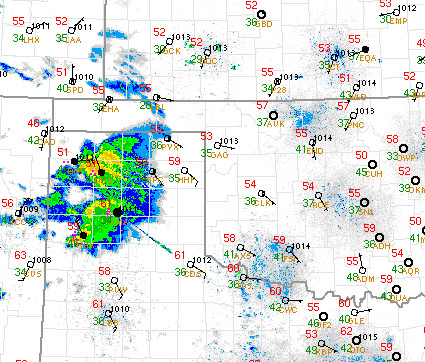[--MAIN HOME--] [--ALL HABYHINTS--] [--FACEBOOK PAGE--]

 DEWPOINT DEPRESSION

METEOROLOGIST JEFF HABY

The dewpoint depression is the difference between the temperature and the dewpoint. It is found by taking the temperature and subtracting the dewpoint. The number that results is a positive number (or 0 if air is saturated). This number that is left is not a temperature but is actually a temperature difference. This can be explained by an example. Suppose the temperature is 50 F with a dewpoint of 40 F. The temperature will always be greater than or equal to the dewpoint. To get the dewpoint depression, the dewpoint of 40 F is subtracted from the temperature value of 50. (50 – 40) = 10. The 10 is the number of Fahrenheit degrees separating the temperature and dewpoint. Note that the 10 is not a temperature, such as 10 F.. but is best labeled as a temperature difference which can be expressed as 10 units of F difference.

The dewpoint depression helps give an idea how close the air is to saturation. In saturated or nearly saturated air the dewpoint depression will be 5 units of F difference or less. As the dewpoint depression gets larger, then the air feels drier and the relative humidity decreases. Large dewpoint depressions can occur on dry days in arid and semi-arid locations. For example, a 100 F temperature with a dewpoint of 30 F results in a dewpoint depression of 70 units of F difference. This is very dry air with a very low relative humidity.

The image below shows several station plots. Many of the observations have temperatures in the 50s with dewpoints in the 30s and 40s. For example, in the Oklahoma City area the temperature is 54 F with a dewpoint of 36 F. This results in a dewpoint depression of 18 units of F difference. This results in a relative humidity in the vicinity of 50%. Note the storms in the Texas panhandle. With relatively dry air near the surface it can be expected that strong evaporative cooling will take place for locations that experience rain. This cooling and cool downdraft air from the storm will result in significant cooling and an increase of the dewpoint due to the evaporation of moisture into the air.